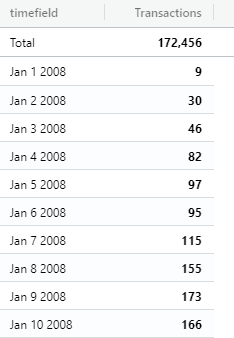# Specify granularity over the time rows in Pivot Table

#1

Hi there, I am working with a pivot table that contains some time fields. The problem is that the query is grouping the rows of those fields with the year as the default granularity. How can I specify the granularity for a specific row?

The following is an example code of what I have:

``````let provider = cf.provider("Elasticsearch");
let source = provider.source('source_name');
let metric = new cf.Metric("count");
let myData = source
.rows("timefield")
.columns()
.metrics(metric);

let myChart = myData.graph("Pivot Table")
.limit(1000)
.set("autoSizeColumns", true)
.execute();
``````

After execute that, the result is the one bellow:

timefield counts
Total 172,563
2008 172,563

Any guidance or suggestions?

#2

Hi @emma, for this case, chartfactor supports specifying different granularities to an instance of the `Row` class by calling the `func ()` function, and to achieve this you must use it as follows:

``````let myData = source
.rows(cf.Row("timefield").func("YEAR | MONTH | WEEK | DAY | HOUR | MINUTE | SECOND"))
.columns()
.metrics(metric);
``````

As you can see in the previous code, by calling the `func()` function with a specific granularity you can achieve to group any field of type `TIME` by the required format.

Thanks @juan.dominguez , your answer is exactly what I was looking for. With that I can set different granularities according to my needs. Setting the granularity as `DAY` I’ve got this:Nice, thanks a lot!